# Is an Induction Hob Expensive to Run? (Average UK Running Costs in 2021)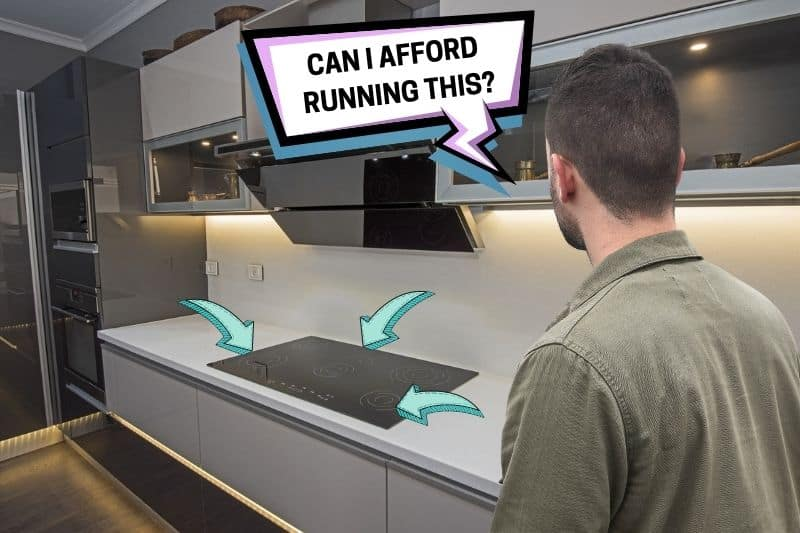Induction hobs are relatively expensive to buy, but are they expensive to run? These are hobs are known for being energy efficient, so you might assume that they would be very cheap to run.

However, the answer isn’t so simple when you compare them to other cooking methods such as gas.

In this article, we’ll look at average induction hob energy usage and running costs.

## How Much Energy Do Induction Hobs Use?

We looked at 10 of the top-selling induction, gas, and ceramic electric hobs in the UK to determine their energy usage.

We found that in terms of wattage, the three hob types are similar:

• Average induction hob ring wattage: 1.95 kW
• Average gas hob ring wattage: 1.95 kW
• Average electric ceramic ring wattage: 1.65 kW

The amount of energy you use will depend on how many rings you use and how long you use them. If you just heat something up for a few minutes on one ring, you will use a lot less energy than you would if you were preparing a complex meal using all four or five hob rings.

## Induction Hob Running Costs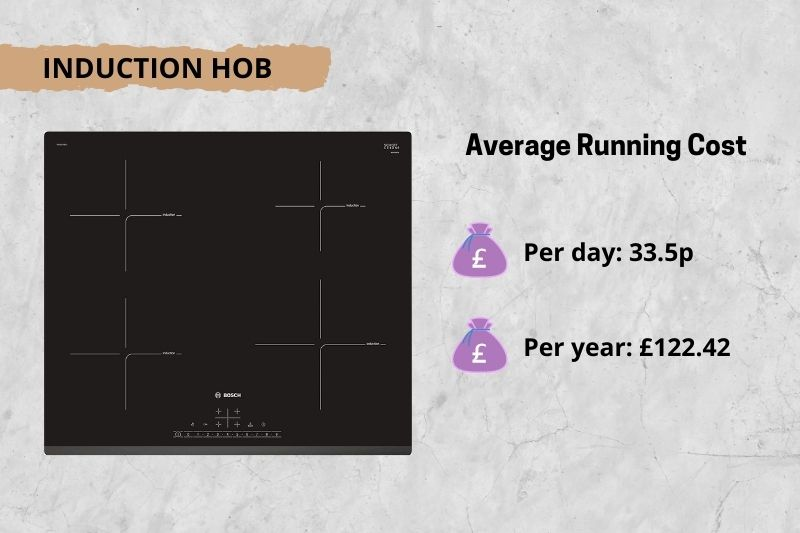The average cost of electricity per kWh in the UK is 17.2p and the average wattage of an induction hob ring is 1.95 kW.

### Per day

If you use an average of one induction ring for one hour a day, the cost of running an induction hob per day would be:

• 17.2p x 1.95 = 33.5p

### Per year

If you use an average of one induction ring for one hour every day of the year, the annual running costs would be:

• 17.2p x 1.95 x 365 = £122.42

## Gas Hob Running Costs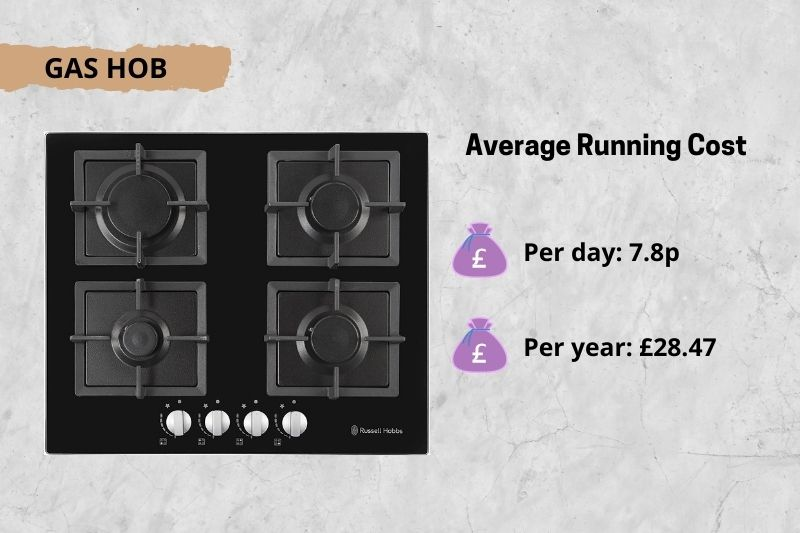The average cost of gas per kWh in the UK is much cheaper than electricity, at around 4p. The average wattage of a gas hob ring is 1.95 kW.

### Per day

If you use an average of one gas hob ring for one hour a day, the cost of running a gas hob per day would be:

• 4 x 1.95 = 7.8p

### Per year

If you use an average of one gas hob ring for one hour every day of the year, the annual running costs would be:

• 4 x 1.95 x 365 = £28.47

## Ceramic Hob Running Costs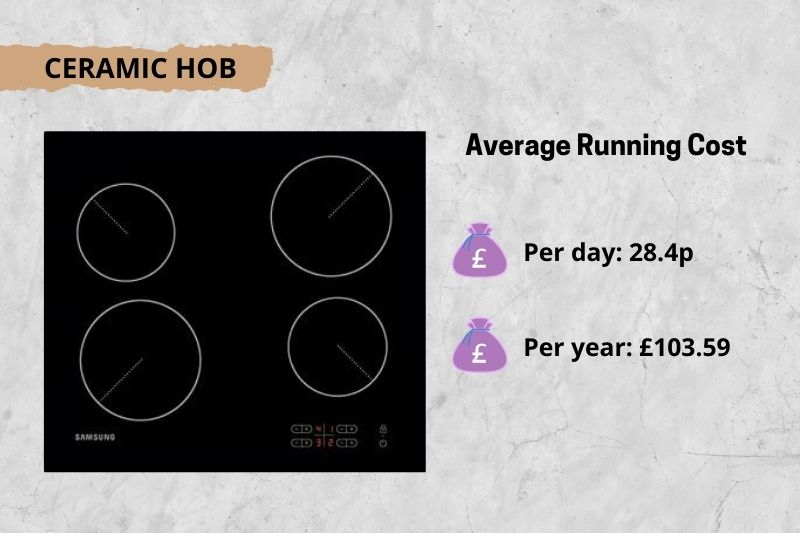The average cost of electricity per kWh in the UK is 17.2p and the average wattage of a ceramic electric hob ring is 1.65 kW.

### Per day

If you use an average of one ceramic hob ring for one hour a day, the cost of running a gas hob per day would be:

• 17.2 x 1.65 = 28.4p

### Per year

If you use an average of ceramic hob ring for one hour every day of the year, the annual running costs would be:

• 17.2 x 1.65 x 365 = £103.59

## Is Induction Really More Expensive to Run?

Based on the figures above, an induction hob is about four times more expensive to run per hour than a gas hob. However, because induction hobs are so much more energy-efficient, you won’t need to leave it on as long, so it won’t be quite as expensive compared to gas.

When the energy efficiency of induction is taken into account, we estimate that running costs will be roughly twice those of a gas hob.

Induction hobs work out cheaper to run than ceramic electric hobs since they are much more efficient. This goes some way to making up for the higher up-front cost of buying an induction hob.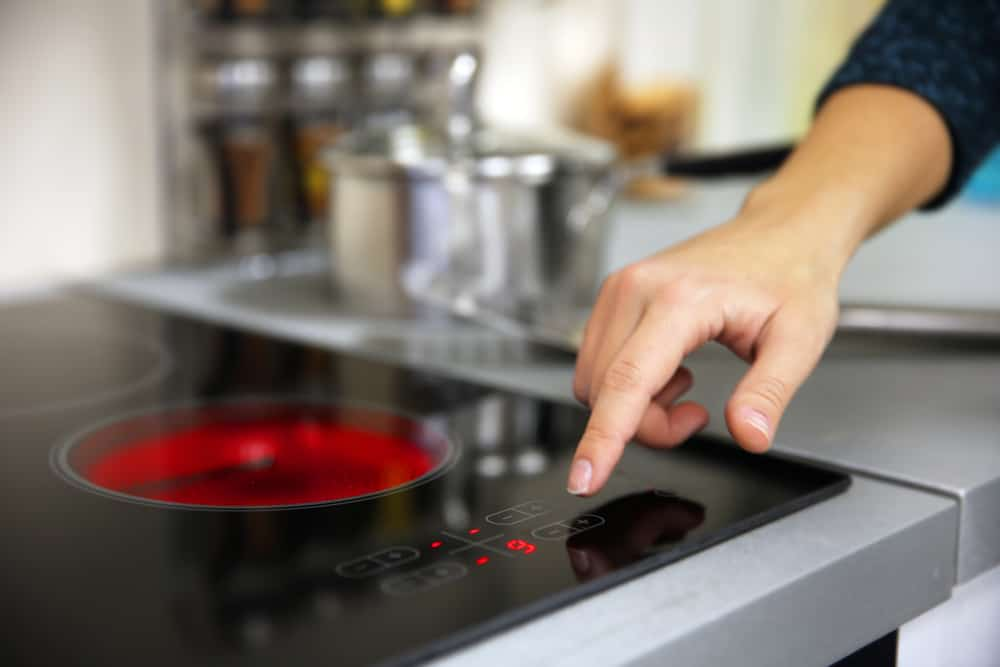This study published by the American Council for an Energy-Efficient Economy (ACEEE) found that with induction cooking, as much as 90% of the energy consumed is transferred to the food, compared to just 40% for gas and 74% for regular electric hobs.

For a practical example, let’s look at how much it would cost to boil a pan of water, taking into account the efficiency of different hob types.

According to research from Which?, this is how long it takes to boil a large pan of water on the average hob:

• Induction hob: 3.56 mins
• Gas hob: 8.27 minutes
• Electric ceramic hob: 7.38 minutes

This means that induction hobs are about twice as fast as other hob types.

Based on this and the figures given above, the cost of boiling a large pan of water would be as follows for the different hob types:

• Induction hob: 0.06 (hours) x 1.95 (kW) x 17.2p (price per kWh) = 2p
• Gas hob: 0.138 (hours) x 1.95 (kW) x 4p (price per kWh) = 1.08p
• Ceramic hob: 0.123 (hours) x 1.65 (kW) x 17.2p (price per kWh) = 3.5p

As you can see, induction is roughly twice as expensive to run as gas, and ceramic is almost twice as expensive again.

## Cost of Buying an Induction Hob

Induction hobs tend to be more expensive than gas and ceramic electric hobs.

At the time of writing, the most popular induction hobs in the UK cost from around £150 to over £2,000, with a median price of about £500.

Gas hobs are cheaper to buy, starting at around £80 and rarely costing more than £500. The median price of a gas hob is around £200.

Electric ceramic hobs are priced somewhere in the middle, costing from about £120 to £700, with a median price of around £250

If you cook a lot, it will be worth investing in an induction hob rather than a ceramic hob, as the energy savings should pay for themselves well before it’s time to replace the hob. You’ll also be able to enjoy the greater responsiveness and speed of induction cooking.

However, if you only cook occasionally and are on a budget, a ceramic hob might be a better choice. Gas remains the cheapest option.